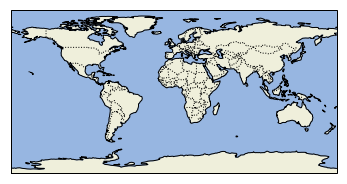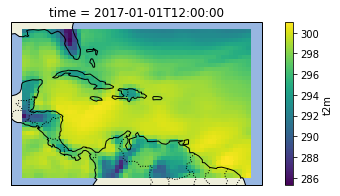# Matplotlib animations from ECMWF data

In our previous post, we saw how to retrieve data from the ECMWF. Today we're going to work on a NetCDF dataset from ERA Interim providing the 2 metre temperature in kelvins. We retrieved this file using this Python code:

from ecmwfapi import ECMWFDataServer
server = ECMWFDataServer()
server.retrieve({
"class": "ei",
"dataset": "interim",
"date": "2017-01-01/to/2017-12-31",
"expver": "1",
"grid": "0.75/0.75",
"levtype": "sfc",
"param": "167.128", # 2 metre temperature
"step": "0",
"stream": "oper",
"time": "00:00:00/06:00:00/12:00:00/18:00:00",
"type": "an",
"format": "netcdf",
"target": "temperature_ei_an_2017.nc",
})


Once we have this data, it's fairly easy to read it with xarray and plot it at a given time using Matplotlib and its Cartopy extension. First we need a few import statements to be able to use these libraries.

In :
import xarray as xr
import matplotlib.pyplot as plt
import cartopy.crs as ccrs
import cartopy.feature as cfeat


Now we can open the NetCDF file using xarray.

In :
ds = xr.open_mfdataset("data/temperature_ei_an_2017.nc")


## Plotting data on a map¶

Once we have this xarray dataset object, we can use it to plot data on a map. First we define a function that will create the base map on which we're going to plot our data.

In :
def make_figure():
fig = plt.figure(figsize=(8, 3))
ax = fig.add_subplot(1, 1, 1, projection=ccrs.PlateCarree())

# generate a basemap with country borders, oceans and coastlines
return fig, ax

make_figure();For this tutorial, we restrict the domain to the carribean area because generating animations on a large domain can be quite ressource intensive. Let's retrieve the 2 metre temperature for this area.

In :
area = ds.t2m.sel(longitude=slice(270, 310), latitude=slice(30, 4))


Then we plot the temperature for this domain on the 1st of January 2017 at 12:00.

In :
_, ax = make_figure()
# plot the temperature field
grid = area.sel(time='2017-01-01T12:00:00')
grid.plot(ax=ax, transform=ccrs.PlateCarree());## Generating an animated map¶

As you can see, Cartopy makes it very easy to add a simple basemap that will put your data in context. Now, using Matplotlib's animation module, we can visualize how the temperature changes over time. Note how we draw the color bar only for the initial frame (add_colorbar=True), setting its scale based on the data.

In :
import matplotlib.animation as animation

fig, ax = make_figure()

frames = area.time.size        # Number of frames
min_value = area.values.min()  # Lowest value
max_value = area.values.max()  # Highest value

grid = area[frame]
contour = grid.plot(ax=ax, transform=ccrs.PlateCarree(),
title = u"%s — %s" % (ds.t2m.long_name, str(area.time[frame].values)[:19])
ax.set_title(title)
return contour

def init():

def animate(frame):